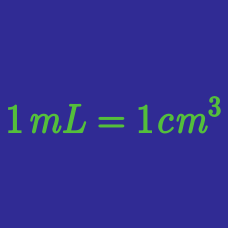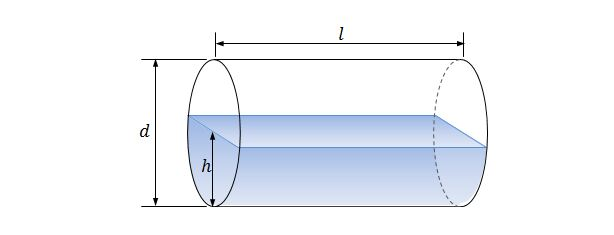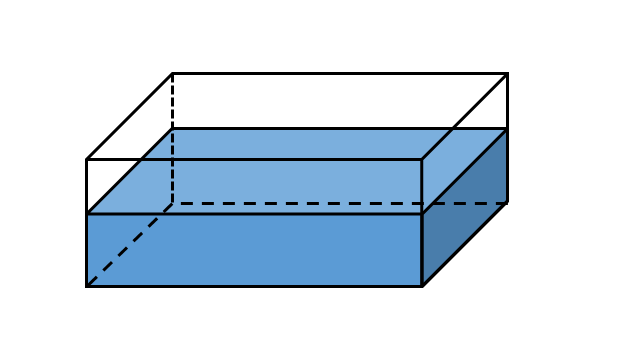Classical Mechanics

# SI Fluid Measure

If a cylindrical container has a base area of $12 \text{ cm}^2$ and a height of $6 \text{ cm}$, how many mL of water can it hold?Liquefied natural gas is filled in a horizontal cylindrical tank, as shown above. The diameter of the circular base and the length of the cylindrical tank are $d=6\text{ m}$ and $l=14\text{ m},$ respectively, and the height of the liquefied natural gas is $h=3\text{ m}.$ Find the volume of the liquefied natural gas in the tank.

$18 0\text{ mL}$ of water is poured into a cylindrical cup with base area $30\text{ cm}^2$ and height $15 \text{ cm}.$ What is the height of the water inside the cup?In the figure above, a water reservoir has a rectangular base floor with dimensions $3\text{ km}\times 2\text{ km}$ and a depth of $8 \text{ m}.$ If this reservoir is half-full, what is the volume of the water in liters?

What is $8\text{ L}$ in $\text{m}^3?$

×